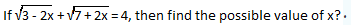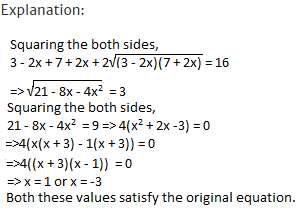## 10 Questions MCQ Test IBPS PO Prelims- Study Material, Online Tests, Previous Year | Test: Quadratic Equations- 2

Description
Attempt Test: Quadratic Equations- 2 | 10 questions in 10 minutes | Mock test for Banking Exams preparation | Free important questions MCQ to study IBPS PO Prelims- Study Material, Online Tests, Previous Year for Banking Exams Exam | Download free PDF with solutions
QUESTION: 1

### Find the value of a/b + b/a, if a and b are the roots of the quadratic equation x2 + 8x + 4 = 0?

Solution:

Explanation:

a/b + b/a = (a2 + b2)/ab = (a2 + b2 + a + b)/ab
= [(a + b)2 - 2ab]/ab
a + b = -8/1 = -8
ab = 4/1 = 4
Hence a/b + b/a = [(-8)2 - 2(4)]/4 = 56/4 = 14.

QUESTION: 2

###Solution:QUESTION: 3

### Find the quadratic equations whose roots are the reciprocals of the roots of 2x2 + 5x + 3 = 0?

Solution:

Explanation:

The quadratic equation whose roots are reciprocal of 2x2 + 5x + 3 = 0 can be obtained by replacing x by 1/x.
Hence, 2(1/x)2 + 5(1/x) + 3 = 0
=> 3x2 + 5x + 2 = 0

QUESTION: 4

A man could buy a certain number of notebooks for Rs.300. If each notebook cost is Rs.5 more, he could have bought 10 notebooks less for the same amount. Find the price of each notebook?

Solution:

Explanation:

Let the price of each note book be Rs.x.
Let the number of note books which can be brought for Rs.300 each at a price of Rs.x be y.
Hence xy = 300
=> y = 300/x
(x + 5)(y - 10) = 300 => xy + 5y - 10x - 50 = xy
=>5(300/x) - 10x - 50 = 0 => -150 + x2 + 5x = 0
multiplying both sides by -1/10x
=> x2 + 15x - 10x - 150 = 0
=> x(x + 15) - 10(x + 15) = 0
=> x = 10 or -15
As x>0, x = 10.

QUESTION: 5

I. a2 - 7a + 12 = 0,
II. b2 - 3b + 2 = 0 to solve both the equations to find the values of a and b?

Solution:

Explanation:

I.(a - 3)(a - 4) = 0
=> a = 3, 4

II. (b - 2)(b - 1) = 0
=> b = 1, 2
=> a > b

QUESTION: 6

I. a2 - 9a + 20 = 0,
II. 2b2 - 5b - 12 = 0 to solve both the equations to find the values of a and b?

Solution:

Explanation:

I. (a - 5)(a - 4) = 0
=> a = 5, 4
II. (2b + 3)(b - 4) = 0
=> b = 4, -3/2 => a ≥ b

QUESTION: 7

I. a2 + 11a + 30 = 0,
II. b2 + 6b + 5 = 0 to solve both the equations to find the values of a and b?

Solution:

Explanation:

I. (a + 6)(a + 5) = 0
=> a = -6, -5
II. (b + 5)(b + 1) = 0
=> b = -5, -1 => a ≤ b

QUESTION: 8

I. a2 + 8a + 16 = 0,
II. b2 - 4b + 3 = 0 to solve both the equations to find the values of a and b?

Solution:

Explanation:

I. (a + 4)2 = 0 => a = -4
II.(b - 3)(b - 1) = 0
=> b = 1, 3 => a < b

QUESTION: 9

I. a2 - 2a - 8 = 0,
II. b2 = 9 to solve both the equations to find the values of a and b?

Solution:

Explanation:

I. (a - 4)(a + 2) = 0
=> a = 4, -2
II. b2 = 9
=> b = ± 3
-2 < 3, -2 > -3, 4 > 3, 4 > -3,
No relation can be established between a and b.

QUESTION: 10

I. x2 + 5x + 6 = 0,
II. y2 + 9y +14 = 0 to solve both the equations to find the values of x and y?

Solution:

I. x2 + 3x + 2x + 6 = 0
=> (x + 3)(x + 2) = 0 => x = -3 or -2
II. y2 + 7y + 2y + 14 = 0
=> (y + 7)(y + 2) = 0 => y = -7 or -2
No relationship can be established between x and y.Use Code STAYHOME200 and get INR 200 additional OFF Use Coupon Code